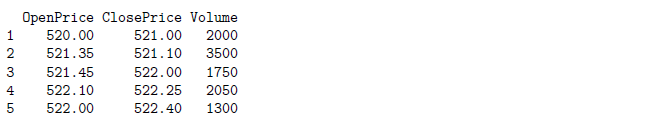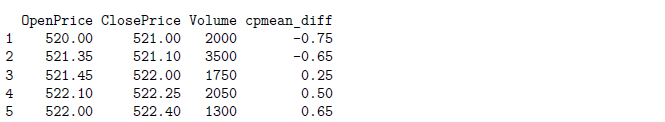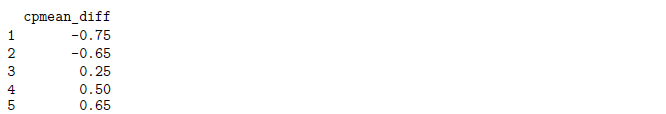# R Weekly Bulletin Vol – XIThis week’s R bulletin will cover topics on how to round to the nearest desired number, converting and comparing dates and how to remove last x characters from an element. Hope you like this R weekly bulletin. Enjoy reading!

### Shortcut Keys

1. Comment/uncomment current line/selection - Ctrl+Shift+C
2. Move Lines Up/Down - Alt+Up/Down
3. Delete Line - Ctrl+D

### Problem Solving Ideas

#### Rounding to the nearest desired number

Consider a case where you want to round a given number to the nearest 25. This can be done in the following manner:

round(145/25) * 25

 150

floor(145/25) * 25

 125

ceiling(145/25) * 25

 150

Usage: Assume if you are calculating a stop loss or take profit for an NSE stock in which the minimum tick is 5 paisa. In such case, we will divide and multiply by 0.05 to achieve the desired outcome.

Example:

Price = 566
Stop_loss = 1/100# without rounding
SL = Price * Stop_loss
print(SL)

 5.66

# with rounding to the nearest 0.05
SL1 = floor((Price * Stop_loss)/0.05) * 0.05
print(SL1)

 5.65

#### How to remove last n characters from every element

To remove the last n characters we will use the substr function along with the nchr function. The example below illustrates the way to do it.

Example:

# In this case, we just want to retain the ticker name which is "TECHM"
symbol = "TECHM.EQ-NSE"
s = substr(symbol,1,nchar(symbol)-7)
print(s)

 "TECHM"

#### Converting and Comparing dates in different formats

When we pull stock data from Google finance the date appears as “YYYYMMDD”, which is not recognized as a date-time object. To convert it into a date-time object we can use the “ymd” function from the lubridate package.

Example:

library(lubridate)
x = ymd(20160724)
print(x)

 "2016-07-24"

Another data provider gives stock data which has the date-time object in the American format (mm/dd/yyyy). When we read the file, the date-time column is read as a character. We need to convert this into a date-time object. We can convert it using the as.Date function and by specifying the format.

dt = "07/24/2016"
y = as.Date(dt, format = "%m/%d/%Y")
print(y)

 "2016-07-24"

# Comparing the two date-time objects (from Google Finance and the data provider) after conversion
identical(x, y)

 TRUE

### Functions Demystified

rank function

The rank function returns the sample ranks of the values in a vector. Ties (i.e., equal values) and missing values can be handled in several ways.

rank(x, na.last = TRUE, ties.method = c("average", "first", "random", "max", "min"))

where, x: numeric, complex, character or logical vector na.last: for controlling the treatment of NAs. If TRUE, missing values in the data are put last; if FALSE, they are put first; if NA, they are removed; if “keep” they are kept with rank NA ties.method: a character string specifying how ties are treated

Examples:

x <- c(3, 5, 1, -4, NA, Inf, 90, 43)
rank(x)

 3 4 2 1 8 7 6 5

rank(x, na.last = FALSE)

 4 5 3 2 1 8 7 6

#### mutate and transmute functions

The mutate and transmute functions are part of the dplyr package. The mutate function computes new variables using the existing variables of a given data frame. The new variables are added to the existing data frame. On the other hand, the transmute function creates these new variables as a separate data frame.

Consider the data frame “df” given in the example below. Suppose we have 5 observations of 1-minute price data for a stock, and we want to create a new variable by subtracting the mean from the 1-minute closing prices. It can be done in the following manner using the mutate function.

Example:

library(dplyr)
OpenPrice = c(520, 521.35, 521.45, 522.1, 522)
ClosePrice = c(521, 521.1, 522, 522.25, 522.4)
Volume = c(2000, 3500, 1750, 2050, 1300)
df = data.frame(OpenPrice, ClosePrice, Volume)
print(df)df_new = mutate(df, cpmean_diff = ClosePrice - mean(ClosePrice, na.rm = TRUE))
print(df_new)# If we want the new variable as a separate data frame, we can use the transmute function instead.
df_new = transmute(df, cpmean_diff = ClosePrice - mean(ClosePrice, na.rm = TRUE))
print(df_new)#### set.seed function

The set.seed function helps generate the same sequence of random numbers every time the program runs. It sets the random number generator to a known state. The function takes a single argument which is an integer. One needs to use the same positive integer in order to get the same initial state.

Example:

# Initialize the random number generator to a known state and generate five random numbers
set.seed(100)
runif(5)

 0.30776611 0.25767250 0.55232243 0.05638315 0.46854928

# Reinitialize to the same known state and generate the same five 'random' numbers
set.seed(100)
runif(5)

 0.30776611 0.25767250 0.55232243 0.05638315 0.46854928

### Next Step

We hope you liked this bulletin. In the next weekly bulletin, we will list more interesting ways and methods plus R functions for our readers.

Update

We have noticed that some users are facing challenges while downloading the market data from Yahoo and Google Finance platforms. In case you are looking for an alternative source for market data, you can use Quandl for the same.